# 图像处理 开 闭操作

## Python+OpenCV图像处理之开闭操作

2020-03-23 21:53:42 javastart 阅读数 108
• ###### MATLAB图像处理

MATLAB图像处理课程

40课时 248分钟 5389人学习 李立宗
免费试看

python实现import cv2

def open_demo(image):
print(image.shape)
gray = cv2.cvtColor(image, cv2.COLOR_BGR2GRAY)
ret, binary = cv2.threshold(gray, 0, 255, cv2.THRESH_BINARY | cv2.THRESH_OTSU)
cv2.imshow("binary", binary)
kernel = cv2.getStructuringElement(cv2.MORPH_RECT, (5, 5))
binary = cv2.morphologyEx(binary, cv2.MORPH_OPEN, kernel)
cv2.imshow("open result", binary)

def close_demo(image):
print(image.shape)
gray = cv2.cvtColor(image, cv2.COLOR_BGR2GRAY)
ret, binary = cv2.threshold(gray, 0, 255, cv2.THRESH_BINARY | cv2.THRESH_OTSU)
cv2.imshow("binary", binary)
kernel = cv2.getStructuringElement(cv2.MORPH_RECT, (5, 5))
binary = cv2.morphologyEx(binary, cv2.MORPH_CLOSE, kernel)
cv2.imshow("close result", binary)

if __name__ == "__main__":
cv2.namedWindow("input image",cv2.WINDOW_AUTOSIZE)
cv2.imshow("input image", img)
open_demo(img)
cv2.waitKey(0)
cv2.destroyAllWindows()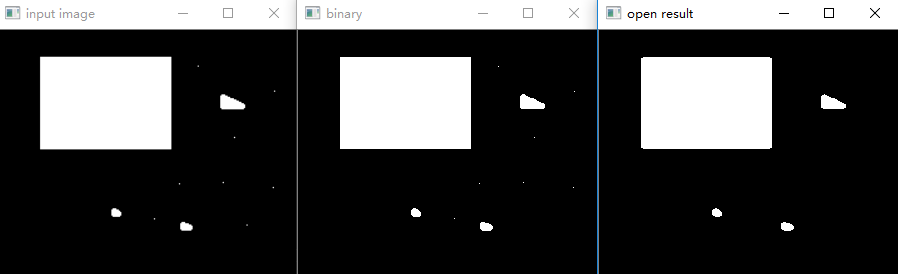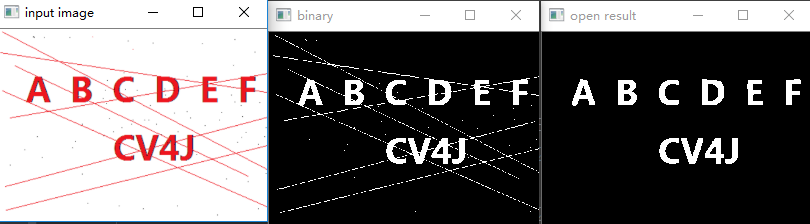kernel = cv2.getStructuringElement(cv2.MORPH_RECT, (15, 1))
binary = cv2.morphologyEx(binary, cv2.MORPH_OPEN, kernel)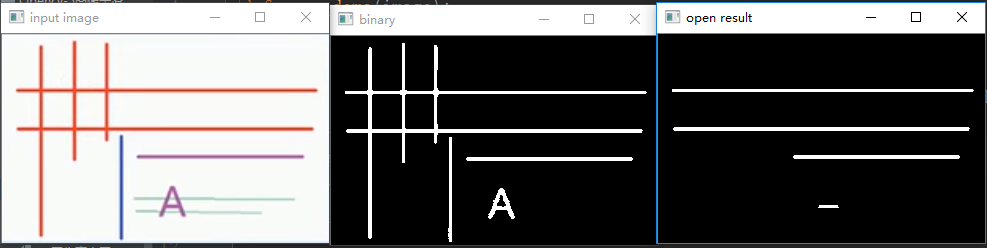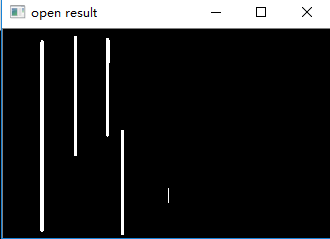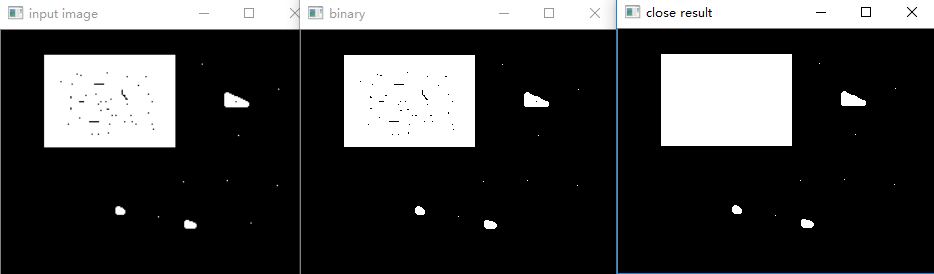kernel = cv2.getStructuringElement(cv2.MORPH_ELLIPSE, (5, 5))
binary = cv2.morphologyEx(binary, cv2.MORPH_CLOSE, kernel)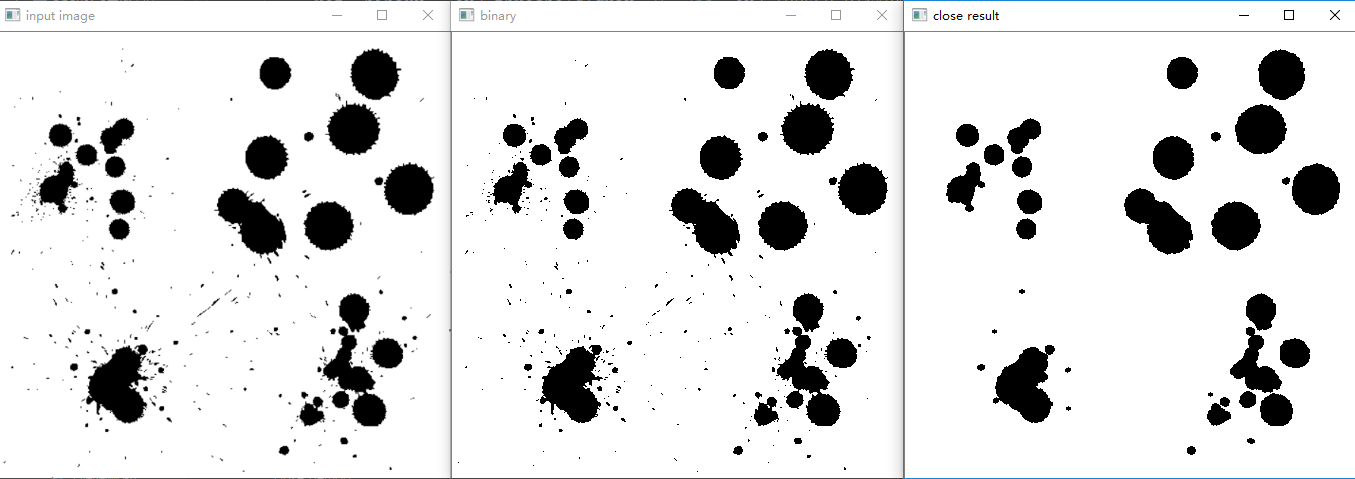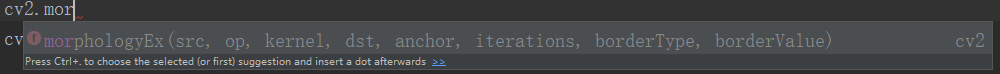src参数：输入图像

op参数：操作类型

MORTH_OPEN                函数做开运算

MORTH_CLOSE              函数做闭运算

MORTH_TOPHAT            函数做顶帽运算

MORTH_BLACKHAT       函数做黑帽运算

MORTH_DILATE              函数做膨胀运算

MORTH_ERODE             函数做腐蚀运算

kernel参数 ：内核类型，用getStructuringElement函数得到

## 数字图像处理之开操作和闭操作

2018-04-21 15:45:00 learning_tortosie 阅读数 14354
• ###### MATLAB图像处理

MATLAB图像处理课程

40课时 248分钟 5389人学习 李立宗
免费试看

# 实验目的

1．理解并掌握形态学图像处理中的开操作和闭操作
2．熟悉并掌握MATLAB软件的使用

# 相关知识

## 1.定义

$A○B=\left(A\ominus B\right)\oplus B$

$A•B=\left(A\oplus B\right)\ominus B$

## 2.几何解释

（1）开操作的何解释
A○B的边界由B中的点建立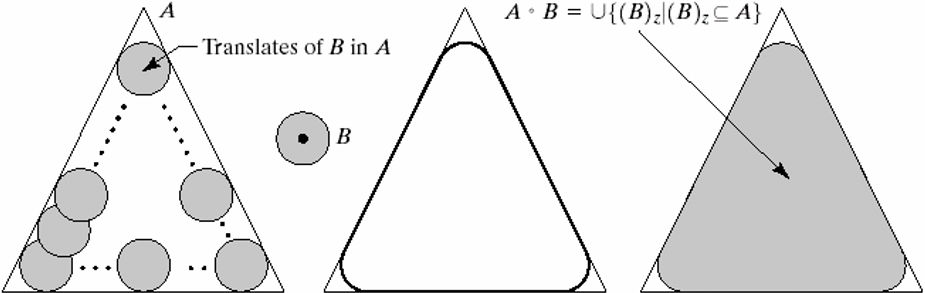（2）闭操作的几何解释
A•B的边界由B中的点建立
B在A的边界外侧滚动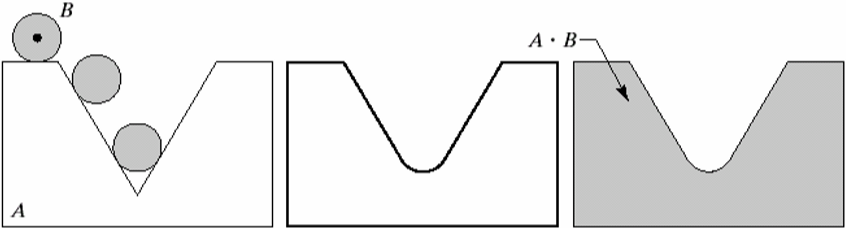## 3.相关函数说明

（1）strel

SE = STREL('arbitrary',NHOOD,HEIGHT) 创建一个指定领域的非平面结构化元素。HEIGHT是一个矩阵，大小和NHOOD相同，他指定了NHOOD中任何非零元素的高度值。
SE = STREL('ball',R,H,N) 创建一个空间椭球状的结构元素，其X-Y平面半径为R，高度为H。R必须为非负整数，H是一个实数。N必须为一个非负偶数。当N>0时此球形结构元素由一系列空间线段结构元素来近似。
SE = STREL('diamond',R) 创建一个指定大小R平面钻石形状的结构化元素。R是从结构化元素原点到其点的距离，必须为非负整数。
SE = STREL('disk',R,N) 创建一个指定半径R的平面圆盘形的结构元素。这里R必须是非负整数. N须是0, 4, 6, 8.当N大于0时,圆盘形结构元素由一组N(或N+2)个周期线结构元素来近似。当N等于0时,不使用近似，即结构元素的所有像素是由到中心像素距离小于等于R的像素组成。N可以被忽略,此时缺省值是4。注: 形态学操作在N>0情况下要快于N=0的情形。

（3）imdilate

# 实验内容

a 图是受噪声污染的指纹的二值图像，噪声为黑色背景上的亮元素和亮指纹部分的暗元素
b图是使用的结构元
c图是使用结构元素对图a腐蚀的结果：背景噪声消除了，指纹中的噪声尺寸增加
d图是使用结构元素对图c膨胀的结果：包含于指纹中的噪声分量的尺寸被减小或被完全消除，带来的问题是：在指纹纹路间产生了新的间断
e图是对图d膨胀的结果，图d的大部分间断被恢复，但指纹的线路变粗了
f图是对图e腐蚀的结果，即对图d中开操作的闭操作。最后结果消除了噪声斑点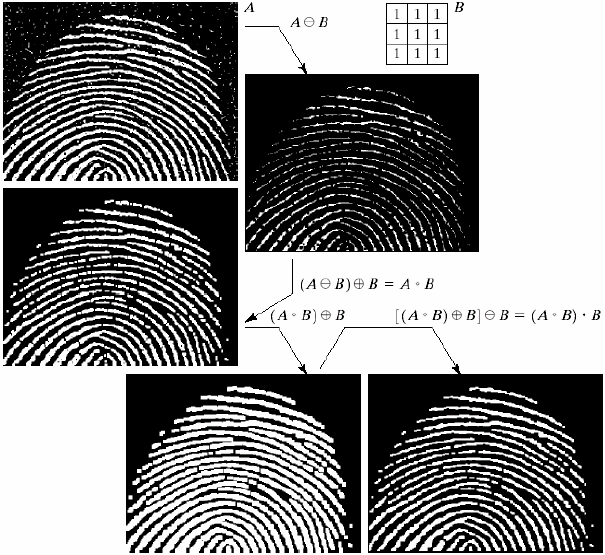# 实验结果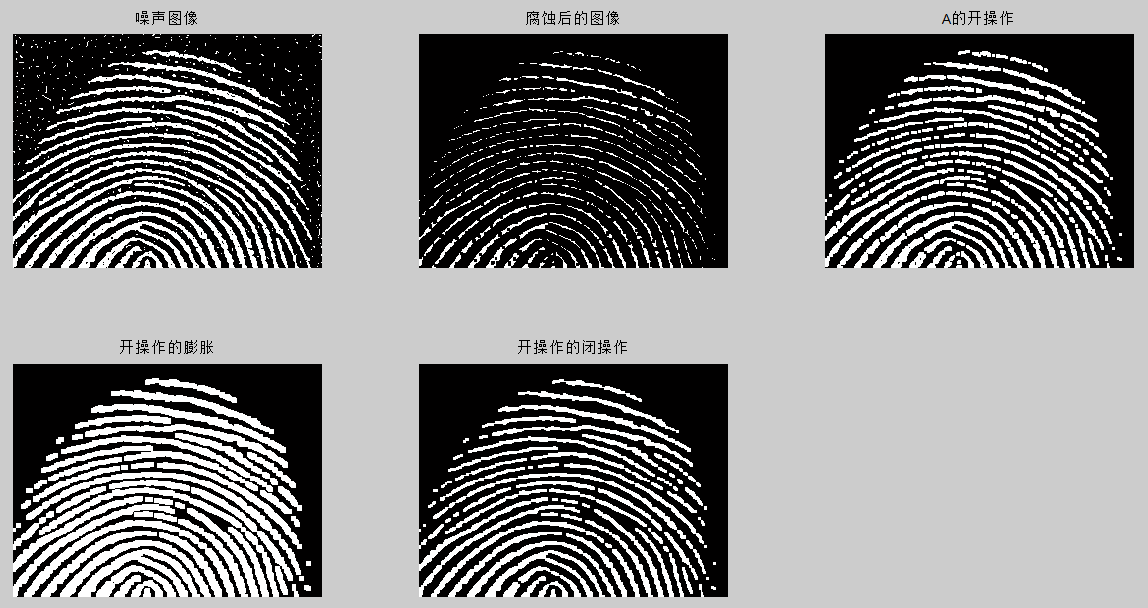# 附录

## matlab程序

A=imread('Fig0911(a)(noisy_fingerprint).tif'); %注意图片的路径要设置正确
subplot(2,3,1);
imshow(A)
title('噪声图像')
se=strel('square',3);
A2=imerode(A,se);
subplot(2,3,2);
imshow(A2)
title('腐蚀后的图像')
A3=imopen(A,se);
subplot(2,3,3);
imshow(A3)
title('A的开操作')
A4=imdilate(A3,se);
subplot(2,3,4);
imshow(A4)
title('开操作的膨胀')
A5=imclose(A3,se);
subplot(2,3,5);
imshow(A5)
title('开操作的闭操作')

## 图像处理之开操作详解

2012-07-01 19:11:14 jia20003 阅读数 11382
• ###### MATLAB图像处理

MATLAB图像处理课程

40课时 248分钟 5389人学习 李立宗
免费试看package com.gloomyfish.morphology;

import java.awt.Color;
import java.awt.image.BufferedImage;

public class OpeningFilter extends BinaryFilter {
private int[][] structure_element;
private Color bgColor;
private Color fgColor;

public OpeningFilter() {
structure_element = new int;
// structure_element = new int;
bgColor = Color.BLACK;
fgColor = Color.WHITE;
}

public void setBackGroundColor(Color c) {
this.bgColor = c;
}

public void setForeGroundColor(Color c) {
this.fgColor = c;
}

public void setElement(int[][] element) {
this.structure_element = element;
}

@Override
public BufferedImage filter(BufferedImage src, BufferedImage dest) {
int width = src.getWidth();
int height = src.getHeight();

if ( dest == null )
dest = createCompatibleDestImage( src, null );

int[] inPixels = new int[width*height];
int[] outPixels = new int[width*height];
src = super.filter(src, null);
getRGB( src, 0, 0, width, height, inPixels );
int index = 0;
int subrow = structure_element.length/2;
int subcol = structure_element.length/2;
int rowoffset = 0, coloffset = 0;
int index2 = 0;
for(int row=0; row<height; row++) {
int ta = 0, tr = 0, tg = 0, tb = 0;
for(int col=0; col<width; col++) {
index = row * width + col;
ta = (inPixels[index] >> 24) & 0xff;
tr = (inPixels[index] >> 16) & 0xff;
tg = (inPixels[index] >> 8) & 0xff;
tb = inPixels[index] & 0xff;
int ta2 = 0, tr2 = 0, tg2= 0, tb2 = 0;
boolean isfound = false;
for(int i=-subrow; i<=subrow; i++) {
for(int j=-subcol; j<=subcol; j++) {
rowoffset = row + i;
coloffset = col + j;
if(rowoffset >=0 && rowoffset < height) {
rowoffset = row + i;
} else {
rowoffset = 0;
}

if(coloffset >= 0 && coloffset < width) {
coloffset = col + j;
} else {
coloffset = 0;
}
index2 = rowoffset * width + coloffset;
ta2 = (inPixels[index2] >> 24) & 0xff;
tr2 = (inPixels[index2] >> 16) & 0xff;
tg2 = (inPixels[index2] >> 8) & 0xff;
tb2 = inPixels[index2] & 0xff;
if(tr2 == bgColor.getRed() && tg2 == bgColor.getGreen()) {
isfound = true;
break;
}
}
if(isfound) break;
}
rowoffset = 0;
coloffset = 0;
if(isfound) {
tr = bgColor.getRed();
tg = bgColor.getGreen();
tb = bgColor.getBlue();
outPixels[index] = (ta << 24) | (tr << 16) | (tg << 8) | tb;
} else {
outPixels[index] = (ta << 24) | (tr << 16) | (tg << 8) | tb;
}

}
}

// copy the Erosion result pixels to input pixels data
// and ready to Dilation operation
System.arraycopy(outPixels, 0, inPixels, 0, width*height);

// start to dilate the pixels data...
for(int row=0; row<height; row++) {
int ta = 0, tr = 0, tg = 0, tb = 0;
for(int col=0; col<width; col++) {
index = row * width + col;
ta = (inPixels[index] >> 24) & 0xff;
tr = (inPixels[index] >> 16) & 0xff;
tg = (inPixels[index] >> 8) & 0xff;
tb = inPixels[index] & 0xff;
int ta2 = 0, tr2 = 0, tg2= 0, tb2 = 0;
boolean isfound = false;
for(int i=-subrow; i<=subrow; i++) {
for(int j=-subcol; j<=subcol; j++) {
rowoffset = row + i;
coloffset = col + j;
if(rowoffset >=0 && rowoffset < height) {
rowoffset = row + i;
} else {
rowoffset = 0;
}

if(coloffset >= 0 && coloffset < width) {
coloffset = col + j;
} else {
coloffset = 0;
}
index2 = rowoffset * width + coloffset;
ta2 = (inPixels[index2] >> 24) & 0xff;
tr2 = (inPixels[index2] >> 16) & 0xff;
tg2 = (inPixels[index2] >> 8) & 0xff;
tb2 = inPixels[index2] & 0xff;
if(tr2 == fgColor.getRed() && tg2 == fgColor.getGreen()) {
isfound = true;
break;
}
}
if(isfound) break;
}
rowoffset = 0;
coloffset = 0;
if(isfound) {
tr = fgColor.getRed();
tg = fgColor.getGreen();
tb = fgColor.getBlue();
outPixels[index] = (ta << 24) | (tr << 16) | (tg << 8) | tb;
} else {
outPixels[index] = (ta << 24) | (tr << 16) | (tg << 8) | tb;
}

}
}

setRGB( dest, 0, 0, width, height, outPixels );
return dest;
}
}


## 图像处理开闭操作(17)

2019-07-15 10:32:00 weixin_30867015 阅读数 113
• ###### MATLAB图像处理

MATLAB图像处理课程

40课时 248分钟 5389人学习 李立宗
免费试看

## 一 定义

1 开操作: 是图像的轮廓变得光滑,断开的较窄的狭颈和消除细的突出物.

使结构元B对集合A进行开操作，定义为：

AB=(AB)B

2 闭操作:同样使图像轮廓变得光滑,但与开操作相反,他能弥合狭窄的间断和细小的沟壑,消除小的空洞,并填补轮廓线中的裂痕.

AB=(AB)B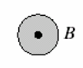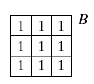## 2 几何解释

1）开操作的何解释
A○B的边界由B中的点建立
当B在A的边界内侧滚动时，B所能到达的A的边界的最远点。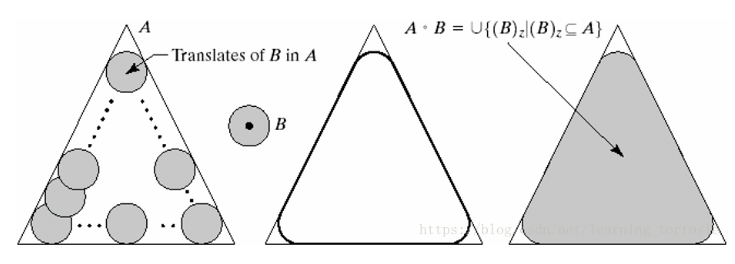(2)闭操作的几何解释

A•B的边界由B中的点建立
B在A的边界外侧滚动
满足〖(B)〗_z⋂A≠”Ø” 的所有点的集合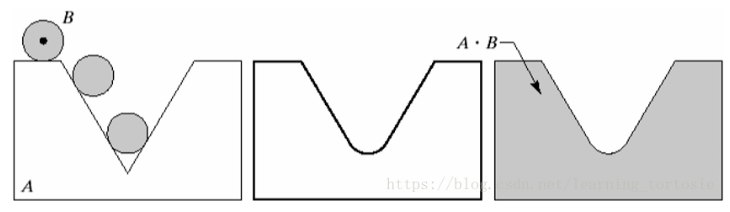## 3 相关函数

### morphologyEx

morphologyEx(   src,
op,
kernel,
dst=None,
anchor=None,
iterations=None,
borderType=None,
borderValue=None
)

.   @param src Source image. The number of channels can be arbitrary. The depth should be one of.   CV_8U, CV_16U, CV_16S, CV_32F or CV_64F.　　　　　　　　　　来源图片。通道数可以是任意的。深度应该是其中之一 。 CV_8U，CV_16U，CV_16S，CV_32F或CV_64F。.   @param op Type of a morphological operation, see #MorphTypes　　　　　　　　形态操作的类型 cv.MORPH_OPEN, cv.MORPH_CLOSE
.   @param kernel Structuring element. It can be created using #getStructuringElement.　　　　　　　　内核(element)
.   @param anchor Anchor position with the kernel. Negative values mean that the anchor is at the.   kernel center.　　　　　　　　用内核锚定位置。负值意味着锚点位于 。核心中心。.   @param iterations Number of times erosion and dilation are applied.　　　　　　　　侵蚀和扩张的次数。.   @param borderType Pixel extrapolation method, see #BorderTypes　　　　　　　　像素外推方法，.   @param borderValue Border value in case of a constant border. The default value has a special.   meaning.　　　　　　　　边界不变的边界值。默认值有一个特殊值 。含义。

### getStructuringElement

形态学处理的核心就是定义结构元素，在OpenCV-Python中，可以使用其自带的getStructuringElement函数，也可以直接使用NumPy的ndarray来定义一个结构元素。首先来看用getStructuringElement函数定义一个结构元素：

element = cv2.getStructuringElement(cv2.MORPH_CROSS,(5,5))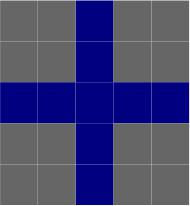NpKernel = np.uint8(np.zeros((5,5)))
for i in range(5):
NpKernel[2, i] = 1 #感谢chenpingjun1990的提醒，现在是正确的
NpKernel[i, 2] = 1

[[0 0 1 0 0]
[0 0 1 0 0]
[1 1 1 1 1]
[0 0 1 0 0]
[0 0 1 0 0]]

用OpenCV-Python内置的常量定义椭圆（MORPH_ELLIPSE）和十字形结构（MORPH_CROSS）元素要简单一些，如果定义矩形（MORPH_RECT）和自定义结构元素，则两者差不多。

## 4 实验结果展示

a 图是受噪声污染的指纹的二值图像，噪声为黑色背景上的亮元素和亮指纹部分的暗元素
b图是使用的结构元
c图是使用结构元素对图a腐蚀的结果：背景噪声消除了，指纹中的噪声尺寸增加
d图是使用结构元素对图c膨胀的结果：包含于指纹中的噪声分量的尺寸被减小或被完全消除，带来的问题是：在指纹纹路间产生了新的间断
e图是对图d膨胀的结果，图d的大部分间断被恢复，但指纹的线路变粗了
f图是对图e腐蚀的结果，即对图d中开操作的闭操作。最后结果消除了噪声斑点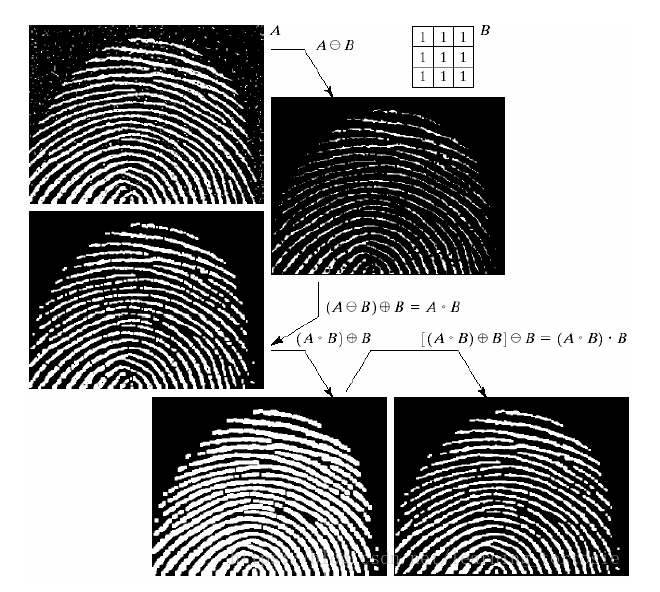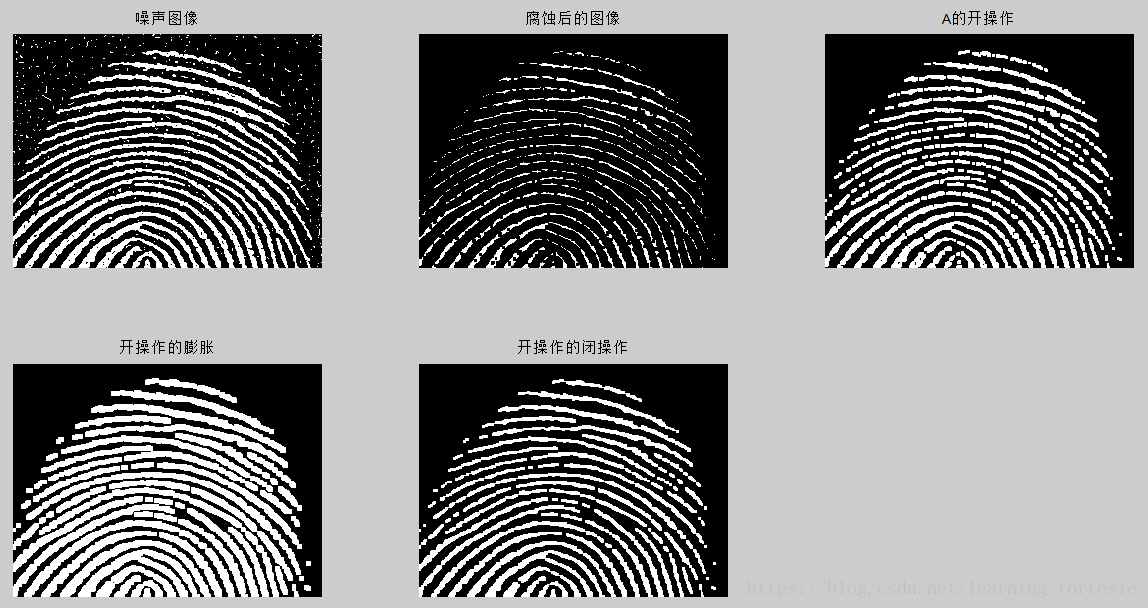## 5 图片腐蚀

# coding=utf-8
import cv2
import numpy as np

# OpenCV定义的结构元素
kernel = cv2.getStructuringElement(cv2.MORPH_RECT, (3, 3))

# 腐蚀图像
eroded = cv2.erode(img, kernel)
# 显示腐蚀后的图像
cv2.imshow("Eroded Image", eroded)

# 膨胀图像
dilated = cv2.dilate(img, kernel)
# 显示膨胀后的图像
cv2.imshow("Dilated Image", dilated)
# 原图像
cv2.imshow("Origin", img)

# NumPy定义的结构元素
NpKernel = np.uint8(np.ones((3, 3)))
Nperoded = cv2.erode(img, NpKernel)
# 显示腐蚀后的图像
cv2.imshow("Eroded by NumPy kernel", Nperoded)

cv2.waitKey(0)
cv2.destroyAllWindows()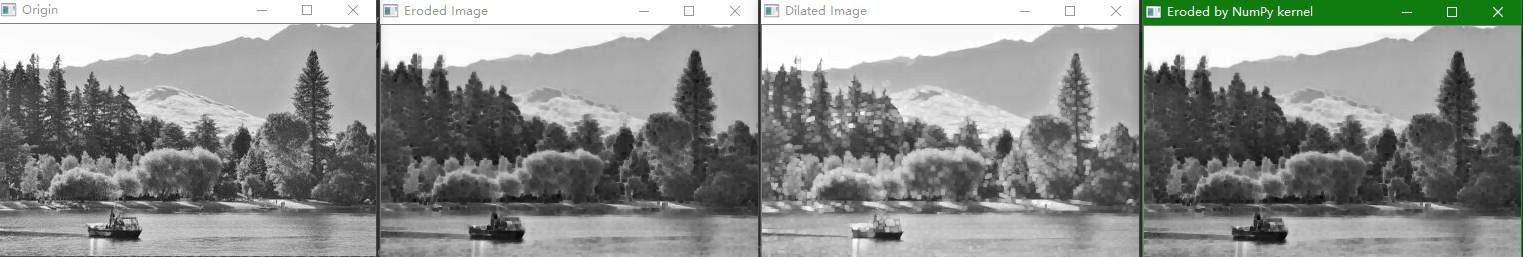## 六 图像的开闭操作

import cv2 as cv

def open_image(image):
'图像开操作'
gray=cv.cvtColor(image,cv.COLOR_BGR2GRAY)
# res=cv.bitwise_not(gray)
#图像二值化
ret,binary=cv.threshold(gray,0,255,cv.THRESH_BINARY | cv.THRESH_OTSU)
cv.imshow('binary',binary)
#获取形态学结构kernel,采用的形态学方式MORPH_RECT
kernel=cv.getStructuringElement(cv.MORPH_RECT,(5,5))
#图像的开操作
binary=cv.morphologyEx(binary,cv.MORPH_OPEN,kernel)
cv.imshow('open',binary)

def close_image(image):
'图像闭操作'
gray=cv.cvtColor(image,cv.COLOR_BGR2GRAY)
# res=cv.bitwise_not(gray)
ret,binary=cv.threshold(gray,0,255,cv.THRESH_BINARY | cv.THRESH_OTSU)
kernel=cv.getStructuringElement(cv.MORPH_RECT,(5,5))
#操作函数morphologyEx
binary=cv.morphologyEx(binary,cv.MORPH_CLOSE,kernel)
cv.imshow('close',binary)

cv.imshow("原来", src)
open_image(src)
close_image(src)
cv.waitKey(0)
cv.destroyAllWindows()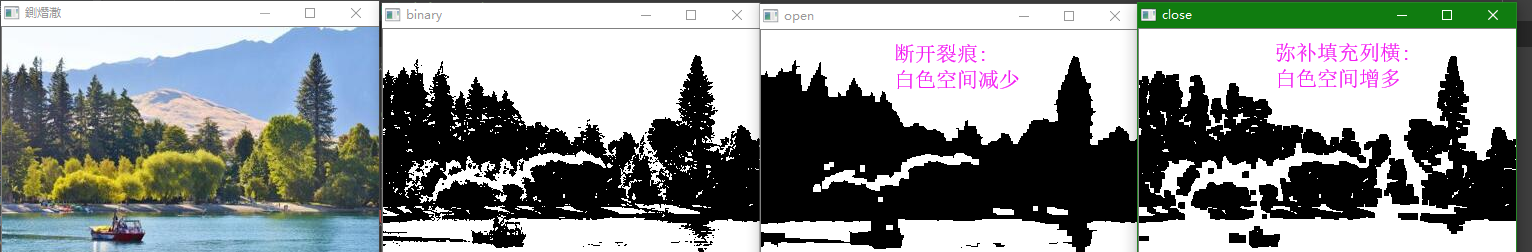闭运算用来连接被误分为许多小块的对象,而开运算用于移除图像噪音形成的斑点.因此，某些情况下可以连续运用这两种运算。如对一副二值图连续使用闭运算和开运算，将获得图像中的主要对象。同样，如果想消除图像中的噪声（即图像中的“小点”），也可以对图像先用开运算后用闭运算，不过这样也会消除一些破碎的对象。

## 图像开和闭操作

2011-05-04 14:52:00 yeqiu712 阅读数 5535
• ###### MATLAB图像处理

MATLAB图像处理课程

40课时 248分钟 5389人学习 李立宗
免费试看